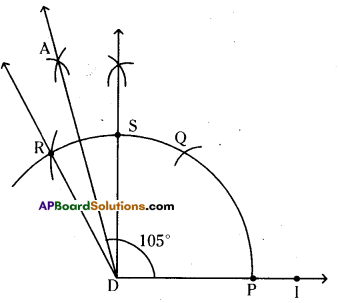AP State Syllabus AP Board 6th Class Maths Solutions Chapter 10 Practical Geometry Ex 10.4 Textbook Questions and Answers.

## AP State Syllabus 6th Class Maths Solutions 10th Lesson Practical Geometry Ex 10.4Question 1.
Construct ∠ABC = 60° without using protractor.
Solution:
Steps of construction :

1. Draw a line l and mark a point B on it.
2. Place the pointer of the compasses at B and draw an arc of convenient radius which cuts the line l at a point C.
3. With the pointer at C as centre and with the same radius as above. Now draw an arc that passes through B.
4. Cut intersecting point of two arcs is A. Join BA. ∠ABC = 60° is formed.
Hence the required angle ∠ABC = 60° is constructed.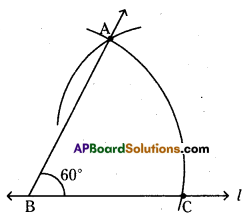Question 2.
Construct an angle of 120° with using protractor and compasses.
Solution:
Steps of construction :

1. Draw a ray $$\overline{\mathrm{OA}}$$ of any length.
2. Place the pointer of the compasses at O. With O as centre and any convenient radius draw an arc cutting OA at M.
3. With M as centre and without altering radius draw an arc which cuts the previous arc at P.
4. With P as centre and without changing radius draw an arc which cuts the first arc at Q.
5. Join OQ. Then ∠AOQ is the required angle.
Hence the required angle ∠AOQ = 120° is constructed.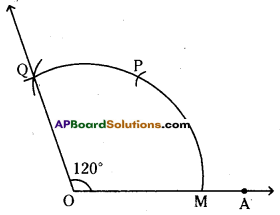Question 3.
Construct the following angles using ruler and compasses. Write the steps of construction in each case.
i) 75°
ii) 15°
iii) 105°
Solution:
i) 75°
Steps of construction:

1. Construct ∠RAM 900
2. Construct ∠PAM = 600 AM as common arm
3. Now, ∠PAR = ∠RAM – ∠PAM = 90° – 60° = 30°
4. Draw bisector to ∠PAR which is $$\overrightarrow{\mathrm{AQ}}$$.
5. Now, ∠MAQ = ∠PAM + ∠PAQ = 60° + $$\frac{30^{\circ}}{2}$$
= 60° + 15° = 75° (QED)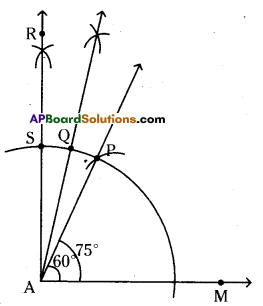ii) 15°
Steps of construction:

1. Construct ∠BQR = 60°
2. Draw the bisector to ∠BQR which is $$\overrightarrow{\mathrm{QC}}$$
3. Now,∠CQR = $$\frac{60^{\circ}}{2}$$ = 30°
4. Draw the bisector $$\overrightarrow{\mathrm{QP}}$$ to∠CQR.
5. ∠PQR = $$\frac{1}{2}$$ x 30° = 15° (Q.E.D)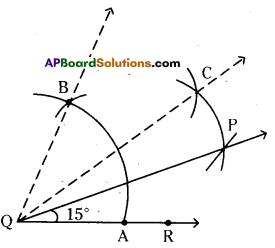iii) 105°
Steps of construction :

1. Construct ∠IDS = 90°
2. Construct ∠ADS = $$\frac{\angle \mathrm{RDS}}{2}=\frac{30^{\circ}}{2}$$ = 15°